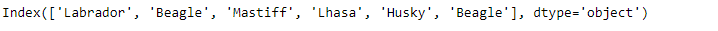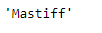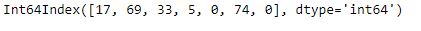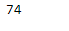# Python | Pandas Index.max()

• Last Updated : 17 Dec, 2018

Python is a great language for doing data analysis, primarily because of the fantastic ecosystem of data-centric python packages. Pandas is one of those packages and makes importing and analyzing data much easier.

Pandas` Index.max()` function returns the maximum value of the Index. The function works with both numerical as well as the string type objects. In case of string type object it returns the string which has highest value in lexicographical order.

Syntax: Index.max()

Parameters : Doesn’t take any parameter.

Returns : scalar: Maximum value.

Example #1: Use `Index.max()` function to find the maximum element in the given Index.

 `# importing pandas as pd``import` `pandas as pd`` ` `# Creating the Index``idx ``=` `pd.Index([``'Labrador'``, ``'Beagle'``, ``'Mastiff'``, ``'Lhasa'``, ``'Husky'``, ``'Beagle'``])`` ` `# Print the Index``idx`

Output :Now we find the max value in the given Index.

 `# return max value.``idx.``max``()`

Output :As we can see in the output, the function has returned ‘Mastiff’ which has the highest lexicographical order among the values present in the Index.

Example #2: Use `Index.max()` function to find the maximum value in the Index.

 `# importing pandas as pd``import` `pandas as pd`` ` `# Creating the Index``idx ``=` `pd.Index([``17``, ``69``, ``33``, ``5``, ``0``, ``74``, ``0``])`` ` `# Print the Datetime Index``idx`

Output :Now we will find the maximum value among the labels of the Index.

 `# the function will return the``# maximum value present in the Index``idx.``max``()`

Output :As we can see in the output, the function has returned 74 which is the largest value among the labels of the Index.

My Personal Notes arrow_drop_up